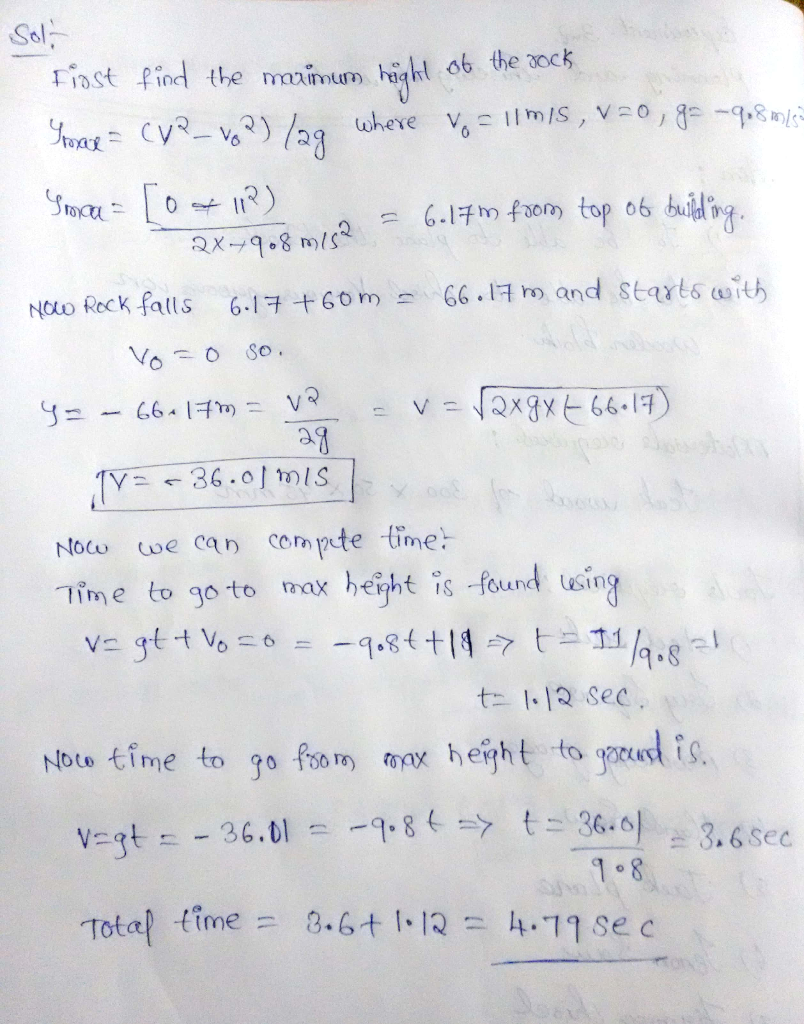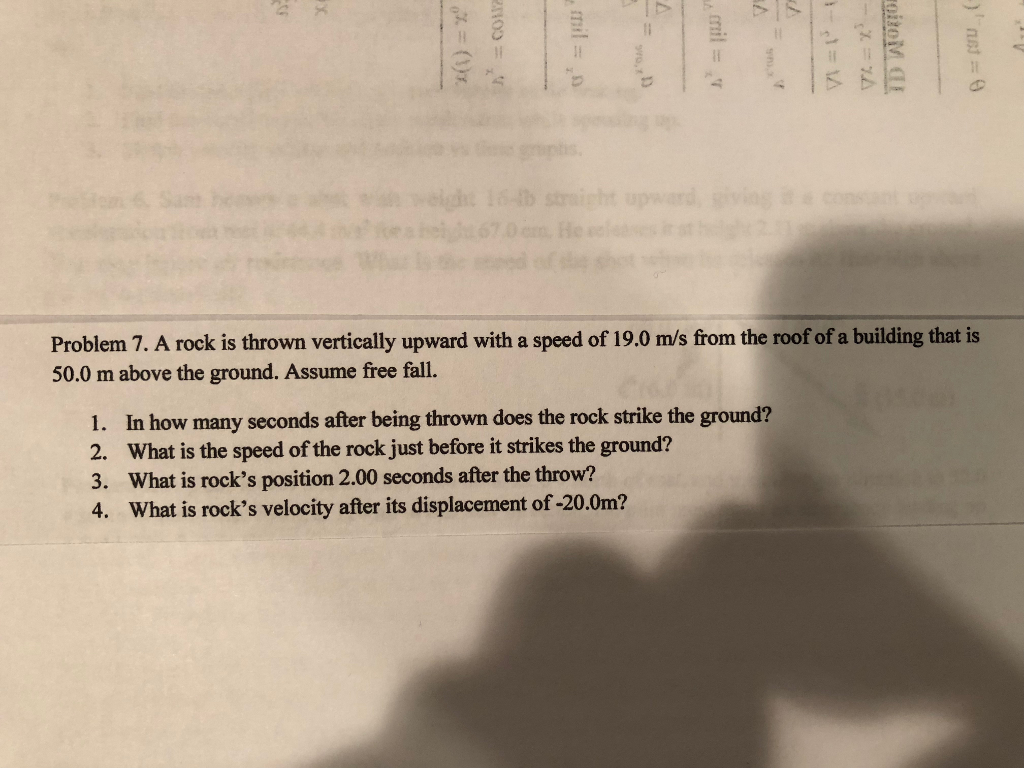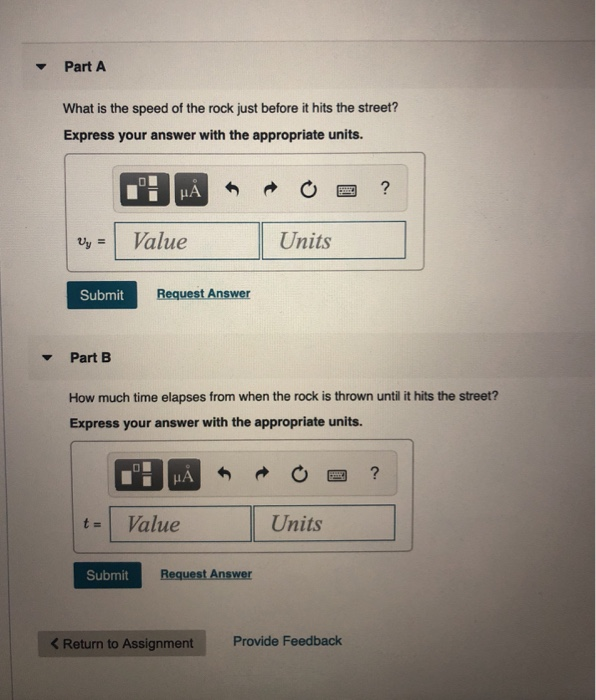# A rock is thrown vertically upward with a speed of 11.0 m/s from the roof of...

A rock is thrown vertically upward with a speed of 11.0 m/s from the roof of a building that is 60.0 mabove the ground. Assume free fall.

1) In how many seconds after being thrown does the rock strike the ground?

2)What is the speed of the rock just before it strikes the ground?#### Earn Coin

Coins can be redeemed for fabulous gifts.

Similar Homework Help Questions
• ### Problem 7. A rock is thrown vertically upward with a speed of 19.0 m/s from the...Problem 7. A rock is thrown vertically upward with a speed of 19.0 m/s from the roof of a building that is 50.0 m above the ground. Assume free fall. 1. In how many seconds after being thrown does the rock strike the ground? 2. What is the speed of the rock just before it strikes the ground? 3. What is rock's position 2.00 seconds after the throw? 4. What is rock's velocity after its displacement of -20.0m?

• ### A rock is thrown upward with a speed of 17.0 m/s from the roof of a building that is 50.0 m...

A rock is thrown vertically upward with a speed of 17.0 m/s from the roof of a building that is 50.0 m above the ground. Assume free fall.In how many seconds after being thrown does the rock strike the ground?

• ### Ch 24-27 HW Problem 2.54 Part A A rock is thrown vertically upward with a speed...Ch 24-27 HW Problem 2.54 Part A A rock is thrown vertically upward with a speed of 17.0 m/s from the roof of a building that is 60.0 m above the ground. Assume free fall. In how many seconds after being thrown does the rock strike the ground AEQ * R O = ? Submit Request Answer Part B What is the speed of the rock just before it strikes the ground? % AXO O ? m/s Uground Submit Request...

• ### A small rock is thrown vertically upward with a speed of 23.0 m/s from the edge...

A small rock is thrown vertically upward with a speed of 23.0 m/s from the edge of the roof of a 39.0-m-tall building. The rock doesn't hit the building on its way back down and lands in the street below. Ignore air resistance. What is the speed of the rock just before it hits the street? How much time elapses from when the rock is thrown until it hits the street?

• ### speed

A rock is thrown vertically upward with a speed of 19.0m/s from the roof of a building that is 50.0m above the ground. Assume free fall.What is the speed of the rock just before it strikes the ground?

• ### A rock is thrown directly upward from the edge of the roof of a building that is 44.5 meters...

A rock is thrown directly upward from the edge of the roof of a building that is 44.5 meters tall. The rock misses the building on its way down, and is observed tostrike the ground 4.00 seconds after being thrown. Take the acceleration due to gravity to have magnitude 9.80 m/s^2 and neglect any effects of air resistance. Withwhat speed was the rock thrown?

• ### A small rock is thrown vertically upward with a speed of 14.0 m/s from the edge...A small rock is thrown vertically upward with a speed of 14.0 m/s from the edge of the roof of a 29.0 m tall building. The rock doesn't hit the building on its way back down and lands in the street below. Air resistance can be neglected. Part A What is the speed of the rock just before it hits the street? Express your answer with the appropriate units. HA ? Uy = Value Units Submit Request Answer Part B...

• ### A ball is thrown vertically upward with a velocity of 15 m/s from the roof of...

A ball is thrown vertically upward with a velocity of 15 m/s from the roof of building that is 22 m high. How long does it take to hit the ground below? How fast was it going when it hit?

• ### a small rock is thrown with a speed of 20m/s and at an angle of 37...

a small rock is thrown with a speed of 20m/s and at an angle of 37 degrees above the horizontal from the roof of a tall building. the rock strikes the grounda distance of 80 m from the base of the building. air resistance can be neglected a)what is the height of the building b)what is the speed of the rock just before it strikes the ground? please show the kinematic equations used

• ### A rock is thrown vertically upward from the ground with an initial speed 15m/s

A rock is thrown vertically upward from the ground with an initial speed 15m/s. a. how high does go? b. how much time is required for the rock to reach its maximum height? c. what is the rock's height at t=2.00s?